# SAT II Chemistry : Avogadro's Number

## Example Questions

### Example Question #1 : Stoichiometry

How many nitrate ions are in one mole of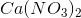?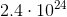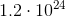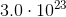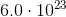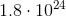Explanation:

Note that there are two nitrate (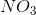) ions for every formula unit of calcium nitrate. This means that in one mole of calcium nitrate, there are two moles of nitrate ions. To go from moles to ions, multiply 2 by Avogadro's number.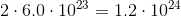### Example Question #1 : Stoichiometry

How many hydrogen atoms are in one mole of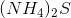?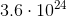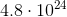Explanation:

In one molecule of, there are 2 ammonium ions, each of which has 4 hydrogen atoms for a total of 8 hydrogen atoms. Thus, to find the number of hydrogen atoms in one mole of, one must multiply Avogadro's number by 8.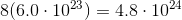### Example Question #1 : Stoichiometry

Calculate the number of aluminum ions inmoles of.Explanation:

In order calculate the number of aluminum ions, we must first find the number of aluminum ions in the entire compound. In this case, there are two molecules offor every molecule of. After understanding this, we can use Avogadro's constant to determine the number of atoms (more specifically ions) of aluminum. See equation below for specific calculations.### Example Question #1 : Avogadro's Number

How many atoms of chloride are in 0.2550 g of aluminum chloride,?Explanation:

The formula for aluminum chloride is. The molar mass ofis 26.98 g and the molar mass ofis 35.45 g. There are threeatoms in. To calculate the molecular mass of, we need to find the sum of the mass of one aluminum atom and three chlorine atoms:The total molecular weight is 130.33 g. Starting with the grams of, we convert to the amount of molesusing the molecular weight value we just calculated. Then we calculate the number of moles of chloride ion in. using the 3:1 ratio. Finally, we calculate the number of atoms by using Avogadro's number. See calculations below: